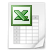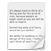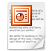### Gaussian Copula R Code Example - JIM ArticleDATA OF THE GAUSSIAN COPULA EXAMPLE
CSV file which inlcude the latent variable scores of the simple corporate reputation model example.
CRP_dataset_std.csv
Comma Separated Value Datei 16.6 KBR CODE OF THE KOLMOGOROV–SMIRNOV TEST WITH LILLIEFORS CORRECTION
This R code allows you to run the Kolmogorov–Smirnov test with Lilliefors correction to assess the non-normality of variables. We use the test to analyze the latent variable scores of COMP, LIKE, and CUSA in the simple corporate reputation model example.
CRP_KS-test_code.r
Text Dokument 852 BytesR CODE OF THE GAUSSIAN COPULA EXAMPLE
This R code allows you to estimate the Gaussian copulas in the structural model of the simple corporate reputation model example. Also, the code returns the bootstrap-based significance of the the Gaussian copulas.
CRP_copula_code.r
Text Dokument 5.3 KBTUTORIAL FOR THE GAUSSIAN COPULA EXAMPLE
This PPT provides additional explanations for the Gaussian copula example on PLS-SEM (i.e., the simple corporate reputation model). Based on these explanations, you should be able to adjust the examples' R code to your own PLS path model.
Endogeneity_Gaussian_Copula_Tutorial.ppt
Microsoft Power Point Präsentation 3.0 MB